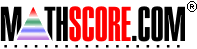Math Practice Online > free > lessons > Florida > 6th grade > Perimeter and Area Word Problems

## Perimeter and Area Word Problems

Word problems with perimeter and area.

 Sample Problems for Perimeter and Area Word Problems Lesson for Perimeter and Area Word Problems

### This topic aligns to the following state standards

Grade 4: Meas 1. Selects an appropriate measurement unit for labeling the solution to real-world problems.
Grade 5: Meas 1. Selects an appropriate measurement unit for labeling the solution to real-world problems.
Grade 4: Geo 1. Compares the concepts of area and perimeter using concrete materials (for example, color tiles, grid paper) and real-world situations (for example, carpeting a floor, fencing a yard).
Grade 5: Geo 2. Applies the concepts of area, perimeter, and volume to solve real-world and mathematical problems using student-developed formulas.
Grade 7: Meas 3. Selects the appropriate unit of measurement when solving real-world problems (for example linear, square, and cubic units).
Grade 6: Meas 1. Selects the appropriate unit of measure for a given real-world situation.
Grade 7: Meas 1. Selects appropriate units of measurement in a real-world context.

Copyright Accurate Learning Systems Corporation 2008.